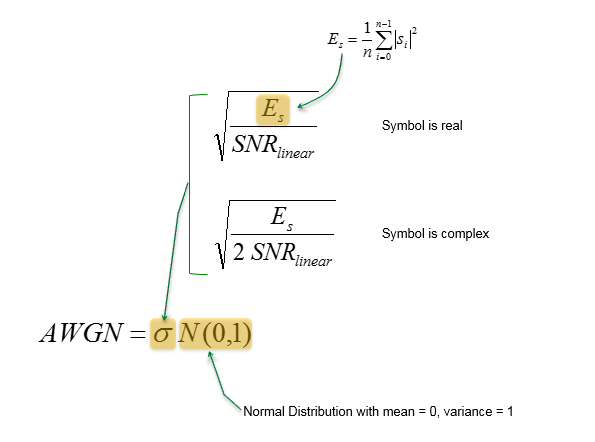Quick Reference - Communication                                  Home : www.sharetechnote.com AWGN, AWGN vs SNR   AWGN stands for Additive WhiteGaussianNoise. Actually this name itself has all the detailed information in it as follows. Additive : This means that the noise is ADDED to the original signal. (Is there any other type (Non-additive) noise ? Yes, for example, you might have heard of multiplicative in some other area like image processing) White : This means that it contains all the frequency components with equal distribution (Is there any other type (non-White)  ? Yes, for example, you might have heard of pink noise (1/f noise) in other area like semiconductor design) Gaussian : This means that it has Gaussian (Normal) Distribution.  (Is there any other type (non-Gaussian) ? Yes, for example, you might have heard of Log Normal Noise)   Some examples of the signal that are impacted by AWGN are as follows.As you see here, the overall distribution of AWGN varies depending on SNR (Signal To Noise Ratio). Following equation shows the relationship between AWGN and SNR.< List 1 >   clear all;   % Number of symbols N = 10000;   % Specify SNR in dB. Try setting various different value here and see how the result changes SNR_dB = 10;   % Create a randomly generated QAM symbols s = (2*randi([0 1],1,N)-1)+j*(2*randi([0 1],1,N)-1);   % Calculate Symbol Energy Eavg = sum(abs(s) .^ 2)/N;   % Convert SNR (in dB) to SNR (in Linear) SNR_lin = 10 .^ (SNR_dB/10);   % Calculate the Sigma (Standard Deviation) of AWGN awgnSigma = sqrt(Eavg/(2*SNR_lin));   % Generate a sequence of noise with Normal Distribution and rescale it with the sigma awgn = awgnSigma*(randn(1,N)+j*randn(1,N));   % Add the noise to the original signal y = s + awgn;   % Plot the noised signal and the original signal plot(real(y),imag(y),'ko','MarkerFaceColor',[0 0 0],'MarkerSize',1); axis([-2 2 -2 2]); title(strcat('SNR=', num2str(SNR_dB), ' dB')); hold on; plot(real(s),imag(s),'ro','MarkerFaceColor',[1 0 0],'MarkerSize',8); axis([-2 2 -2 2]); hold off;   Reference :